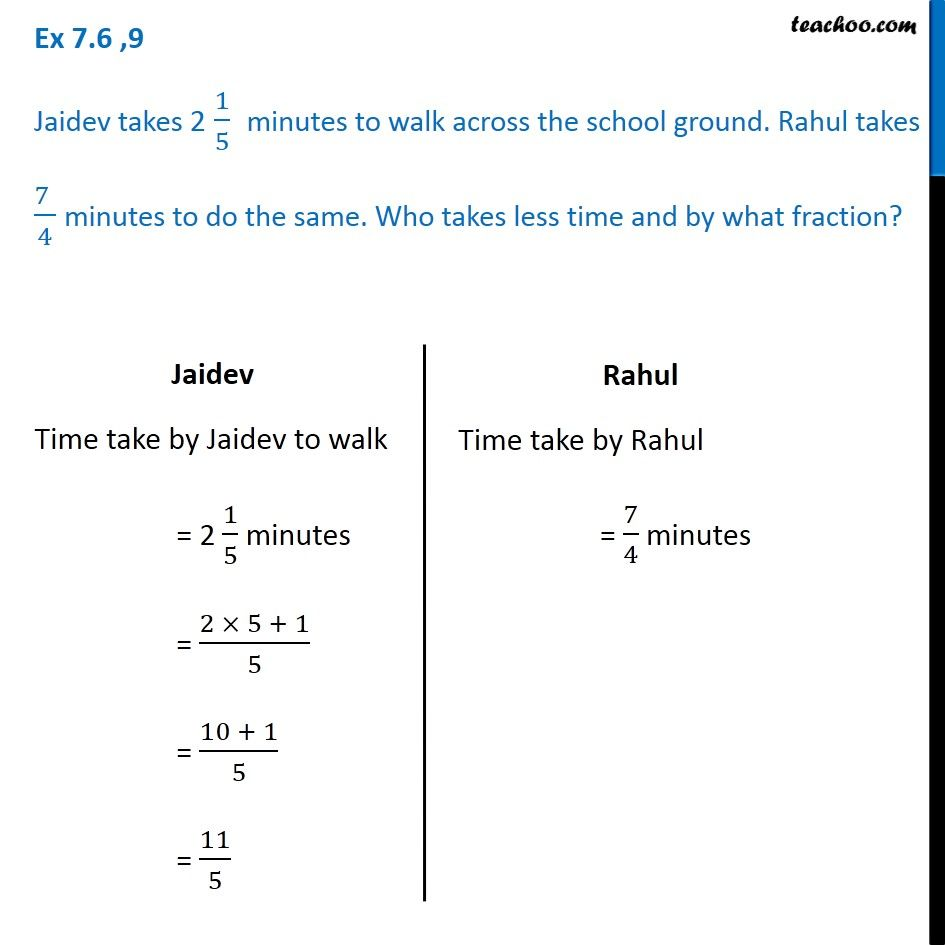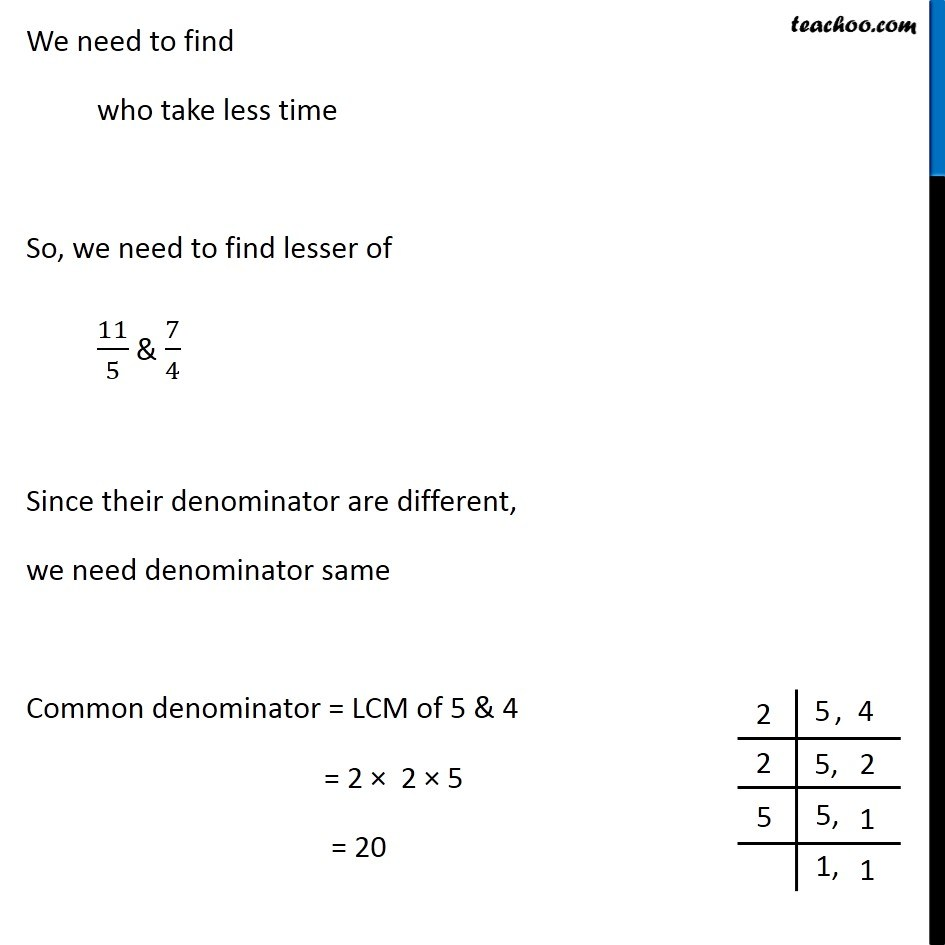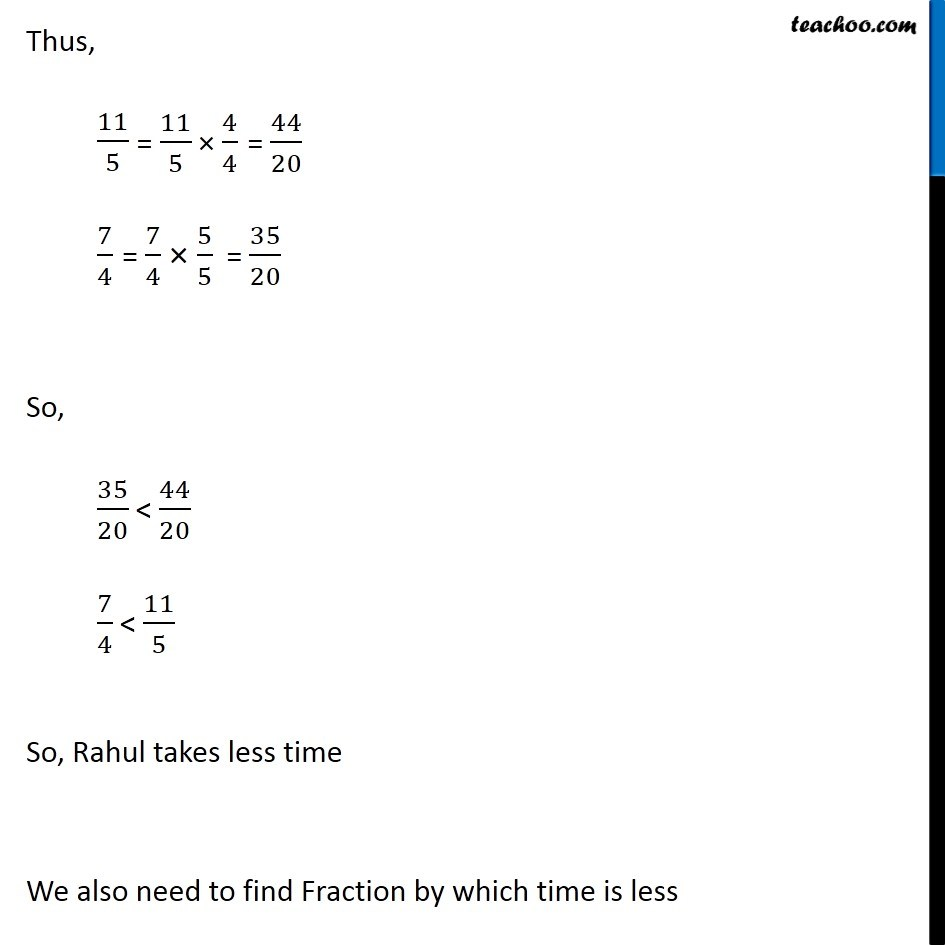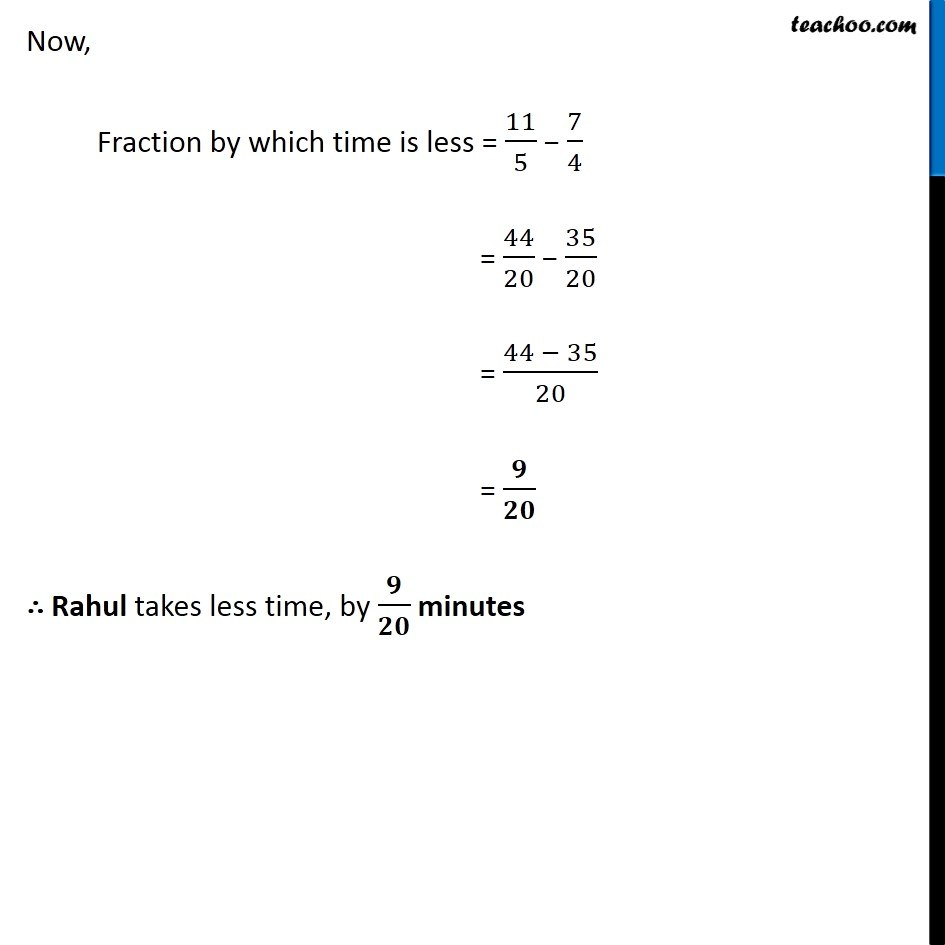1. Chapter 7 Class 6 Fractions
2. Concept wise
3. Statement Questions - Addition and Subtraction of Fractions

Transcript

Ex 7.6 ,9 Jaidev takes 2 1/5 minutes to walk across the school ground. Rahul takes (7 )/4 minutes to do the same. Who takes less time and by what fraction? Jaidev Time take by Jaidev to walk = 2 1/5 minutes = (2 × 5 + 1)/5 = (10 + 1)/5 = 11/5 Rahul Time take by Rahul = 7/4 minutes We need to find who take less time So, we need to find lesser of 11/5 & 7/4 Since their denominator are different, we need denominator same Common denominator = LCM of 5 & 4 = 2 × 2 × 5 = 20 Thus, 11/5 7/4 So, 35/20 < 44/20 7/4 < 11/5 So, Rahul takes less time We also need to find Fraction by which time is less Now, Fraction by which time is less = 11/5 − 7/4 = 44/20 − 35/20 = (44 − 35)/20 = 𝟗/𝟐𝟎 ∴ Rahul takes less time, by 𝟗/𝟐𝟎 minutes